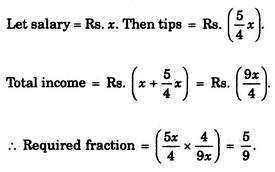# Verbal Reasoning - Arithmetic Reasoning - Discussion

### Discussion :: Arithmetic Reasoning - Section 1 (Q.No.23)

23.

A waiter's salary consists of his salary and tips. During one week his tips were 5/4 of his salary. What fraction of his income came from tips ?

 [A]. 4/9 [B]. 5/4 [C]. 5/8 [D]. 5/9

Explanation:Abinash said: (Jan 19, 2013) The salary word is confusing. The total salary and main salary should be distinguished.

 Kuldeep Bansal said: (Aug 12, 2013) Salary = x. Tip = 5/4. = x+5/4. = 9x/4. Required = 5x/4*4/9x. = 20/36 = 5/9 this answer.

 Mohit said: (Oct 13, 2014) Tip is of 1 week, and salary period is not defined.

 Tisamol Abraham said: (May 8, 2015) I didn't understood this method. How did you got 9x/4? Is there any easier method?

 Ridip Rajkonwar said: (Jun 1, 2015) If the salary be Rs x, then tips = Rs 5x/4. Therefore, total income = salary + tips = x + 5x/4 = (4x+5x) /4 = 9x/4. When total income is 9x/4, tips is 5x/4. When total income is Re 1, tips is (5x/4)/(9x/4) = 5x/4*4/9x = 5/9 (The required fraction).

 Preet Inder said: (Jul 3, 2015) Hey guys tip is given only for a week but time period of salary is not defined. So have a look.

 Anshuli said: (May 6, 2016) If 1:3 is radii, then what will be the area?

 Sneha R said: (Apr 22, 2020) How to calculate the fraction? Could someone please explain.

 Rajkumar said: (Jun 3, 2020) I think in the question the tip is given for only one week. So, (5/4)*x*(4)=5x is the tip for one month. then total income=x+5x=6x, Then required fraction=tip/total income=5x/6x=5/6.

 Athar Ahmed said: (Jul 12, 2020) I couldn't understand. Anyone, please explain me.

 Nita said: (Dec 8, 2020) I think the answer would be 4/9.

 Akash said: (Oct 1, 2021) Yes, right @Rajkumar. 5/6 should be the answer. Salary is given in a month and tips here are weekly in this question.